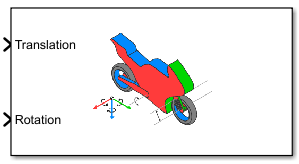Simulation 3D Motorcycle

Implement motorcycle in 3D environment

• Library:
• Vehicle Dynamics Blockset / Vehicle Scenarios / Sim3D / Sim3D Vehicle / Components

•Description

The Simulation 3D Motorcycle block implements a motorcycle with two wheels in the 3D simulation environment.

To use this block, ensure that the Simulation 3D Scene Configuration block is in your model. If you set the Sample time parameter of this block to -1, the block uses the sample time specified in the Simulation 3D Scene Configuration block.

The block input uses the vehicle Z-down right-handed (RH) Cartesian coordinate system defined in SAE J6701. The coordinate system is inertial and initially aligned with the vehicle geometric center:

• X-axis — Along vehicle longitudinal axis, points forward

• Y-axis — Along vehicle lateral axis, points to the right

• Z-axis — Points downward

Tip

Verify that the Simulation 3D Motorcycle block executes before the Simulation 3D Scene Configuration block. That way, Simulation 3D Motorcycle prepares the signal data before the Unreal Engine® 3D visualization environment receives it. To check the block execution order, right-click each block and select Properties. On the General tab, confirm these Priority settings:

• Simulation 3D Scene Configuration0

• Simulation 3D Motorcycle-1

Ports

Input

expand all

Motorcycle and component translation, in m. Array dimensions are 5-by-3.

• Translation(1,1), Translation(1,2), and Translation(1,3) — Motorcycle translation along the inertial vehicle Z-down X-, Y-, and Z- axes, respectively.

• Translation(...,1), Translation(...,2), and Translation(...,3) — Motorcycle component translation relative to vehicle, along the vehicle Z-down X-, Y-, and Z- axes, respectively.

The signal contains translation information according to the locations.

$Translation=\left[\begin{array}{ccc}{X}_{v}& {Y}_{v}& {Z}_{v}\\ {X}_{H}& {Y}_{H}& {Z}_{H}\\ {X}_{SA}& {Y}_{SA}& {Z}_{SA}\\ {X}_{F}& {Y}_{F}& {Z}_{F}\\ {X}_{R}& {Y}_{R}& {Z}_{R}\end{array}\right]$

TranslationArray ElementTranslation Axis

Motorcycle, Xv

Translation(1,1)Inertial vehicle Z-down X-axis

Motorcycle, Yv

Translation(1,2)Inertial vehicle Z-down Y-axis

Motorcycle, Zv

Translation(1,3)Inertial vehicle Z-down Z-axis

Handlebars, XH

Translation(2,1)Vehicle Z-down X-axis

Handlebars, YH

Translation(2,2)Vehicle Z-down Y-axis

Handlebars, ZH

Translation(2,3)Vehicle Z-down Z-axis

Swing arm, XSA

Translation(3,1)Vehicle Z-down X-axis

Swing arm, YSA

Translation(3,2)Vehicle Z-down Y-axis

Swing arm, ZSA

Translation(3,3)Vehicle Z-down Z-axis

Front wheel, XF

Translation(4,1)Vehicle Z-down X-axis

Front wheel, YF

Translation(4,2)Vehicle Z-down Y-axis

Front wheel, ZF

Translation(4,3)Vehicle Z-down Z-axis

Rear wheel, XR

Translation(5,1)Vehicle Z-down X-axis

Rear wheel, YR

Translation(5,2)Vehicle Z-down Y-axis

Rear wheel, ZR

Translation(5,3)Vehicle Z-down Z-axis

Vehicle and component rotation, in rad. Array dimensions are 5-by-3.

• Rotation(1,1), Rotation(1,2), and Rotation(1,3) — Motorcycle rotation about the inertial vehicle Z-down X-, Y-, and Z- axes, respectively.

• Rotation(...,1), Rotation(...,2), and Rotation(...,3) — Motorcycle component rotation relative to vehicle, about the vehicle Z-down X-, Y-, and Z- axes, respectively.

The signal contains rotation information according to the locations.

$Rotation=\left[\begin{array}{ccc}Rol{l}_{v}& Pitc{h}_{v}& Ya{w}_{v}\\ Rol{l}_{H}& Pitc{h}_{H}& Ya{w}_{H}\\ Rol{l}_{SA}& Pitc{h}_{SA}& Ya{w}_{SA}\\ Rol{l}_{F}& Pitc{h}_{F}& Ya{w}_{F}\\ Rol{l}_{R}& Pitc{h}_{R}& Ya{w}_{R}\end{array}\right]$

RotationArray ElementRotation Axis

Vehicle, Rollv

Rotation(1,1)Inertial vehicle Z-down X-axis

Vehicle, Pitchv

Rotation(1,2)Inertial vehicle Z-down Y-axis

Vehicle, Yawv

Rotation(1,3)Inertial vehicle Z-down Z-axis

Handlebar, RollH

Rotation(2,1)Vehicle Z-down X-axis

Handlebar, PitchH

Rotation(2,2)Vehicle Z-down Y-axis

Handlebar, YawH

Rotation(2,3)Vehicle Z-down Z-axis

Swing arm, RollSA

Rotation(3,1)Vehicle Z-down X-axis

Swing arm, PitchSA

Rotation(3,2)Vehicle Z-down Y-axis

Swing arm, YawSA

Rotation(3,3)Vehicle Z-down Z-axis

Front wheel, RollF

Rotation(4,1)Vehicle Z-down X-axis

Front wheel, PitchF

Rotation(4,2)Vehicle Z-down Y-axis

Front wheel, YawF

Rotation(4,3)Vehicle Z-down Z-axis

Rear wheel, RollR

Rotation(5,1)Vehicle Z-down X-axis

Rear wheel, PitchR

Rotation(5,2)Vehicle Z-down Y-axis

Rear wheel, YawR

Rotation(5,3)Vehicle Z-down Z-axis

Parameters

expand all

Vehicle Parameters

Use the Type parameter to specify the motorcycle type. This table provides links to the motorcycle dimensions.

Vehicle Type SettingVehicle Dimensions
Sports bike

Sports Bike

Motor bike

Motor Bike

Scooter

Scooter

Select the color of the vehicle.

Name of motorcycle. By default, when you use the block in your model, the block sets the Name parameter to SimulinkVehicleX. The value of X depends on the number of 3D simulation blocks that you have in your model.

Sample time, Ts. The graphics frame rate is the inverse of the sample time.

Initial Values

Initial motorcycle and component translation, in m. Array dimensions are 5-by-3.

• Translation(1,1), Translation(1,2), and Translation(1,3) — Initial vehicle translation along the inertial vehicle Z-down coordinate system X-, Y-, and Z- axes, respectively.

• Translation(...,1), Translation(...,2), and Translation(...,3) — Initial motorcycle component translation relative to vehicle, along the vehicle Z-down X-, Y-, and Z- axes, respectively.

The parameter contains translation information according to the locations.

$Translation=\left[\begin{array}{ccc}{X}_{v}& {Y}_{v}& {Z}_{v}\\ {X}_{H}& {Y}_{H}& {Z}_{H}\\ {X}_{SA}& {Y}_{SA}& {Z}_{SA}\\ {X}_{F}& {Y}_{F}& {Z}_{F}\\ {X}_{R}& {Y}_{R}& {Z}_{R}\end{array}\right]$

TranslationArray ElementTranslation Axis

Motorcycle, Xv

Translation(1,1)Inertial vehicle Z-down X-axis

Motorcycle, Yv

Translation(1,2)Inertial vehicle Z-down Y-axis

Motorcycle, Zv

Translation(1,3)Inertial vehicle Z-down Z-axis

Handlebars, XH

Translation(2,1)Vehicle Z-down X-axis

Handlebars, YH

Translation(2,2)Vehicle Z-down Y-axis

Handlebars, ZH

Translation(2,3)Vehicle Z-down Z-axis

Swing arm, XSA

Translation(3,1)Vehicle Z-down X-axis

Swing arm, YSA

Translation(3,2)Vehicle Z-down Y-axis

Swing arm, ZSA

Translation(3,3)Vehicle Z-down Z-axis

Front wheel, XF

Translation(4,1)Vehicle Z-down X-axis

Front wheel, YF

Translation(4,2)Vehicle Z-down Y-axis

Front wheel, ZF

Translation(4,3)Vehicle Z-down Z-axis

Rear wheel, XR

Translation(5,1)Vehicle Z-down X-axis

Rear wheel, YR

Translation(5,2)Vehicle Z-down Y-axis

Rear wheel, ZR

Translation(5,3)Vehicle Z-down Z-axis

Initial motorcycle and component rotation, about the vehicle Z-down X-, Y-, and Z- axes.

Array dimensions are 5-by-3.

• Rotation(1,1), Rotation(1,2), and Rotation(1,3) — Initial motorcycle rotation about the inertial vehicle Z-down coordinate systemX-, Y-, and Z- axes, respectively.

• Rotation(...,1), Rotation(...,2), and Rotation(...,3) — Initial motorcycle component rotation relative to vehicle, about the vehicle Z-down X-, Y-, and Z- axes, respectively.

The parameter contains rotation information according to the location.

$Rotation=\left[\begin{array}{ccc}Rol{l}_{v}& Pitc{h}_{v}& Ya{w}_{v}\\ Rol{l}_{FL}& Pitc{h}_{FL}& Ya{w}_{FL}\\ Rol{l}_{FR}& Pitc{h}_{FR}& Ya{w}_{FR}\\ Rol{l}_{RL}& Pitc{h}_{RL}& Ya{w}_{RL}\\ Rol{l}_{RR}& Pitc{h}_{RR}& Ya{w}_{RR}\end{array}\right]$

RotationArray ElementRotation Axis

Vehicle, Rollv

Rotation(1,1)Inertial vehicle Z-down X-axis

Vehicle, Pitchv

Rotation(1,2)Inertial vehicle Z-down Y-axis

Vehicle, Yawv

Rotation(1,3)Inertial vehicle Z-down Z-axis

Handlebar, RollH

Rotation(2,1)Vehicle Z-down X-axis

Handlebar, PitchH

Rotation(2,2)Vehicle Z-down Y-axis

Handlebar, YawH

Rotation(2,3)Vehicle Z-down Z-axis

Swing arm, RollSA

Rotation(3,1)Vehicle Z-down X-axis

Swing arm, PitchSA

Rotation(3,2)Vehicle Z-down Y-axis

Swing arm, YawSA

Rotation(3,3)Vehicle Z-down Z-axis

Front wheel, RollF

Rotation(4,1)Vehicle Z-down X-axis

Front wheel, PitchF

Rotation(4,2)Vehicle Z-down Y-axis

Front wheel, YawF

Rotation(4,3)Vehicle Z-down Z-axis

Rear wheel, RollR

Rotation(5,1)Vehicle Z-down X-axis

Rear wheel, PitchR

Rotation(5,2)Vehicle Z-down Y-axis

Rear wheel, YawR

Rotation(5,3)Vehicle Z-down Z-axis

 Vehicle Dynamics Standards Committee. Vehicle Dynamics Terminology J670. Warrendale, PA: SAE International, 2008.

Vehicle Dynamics Blockset DocumentationGet trial now